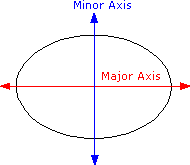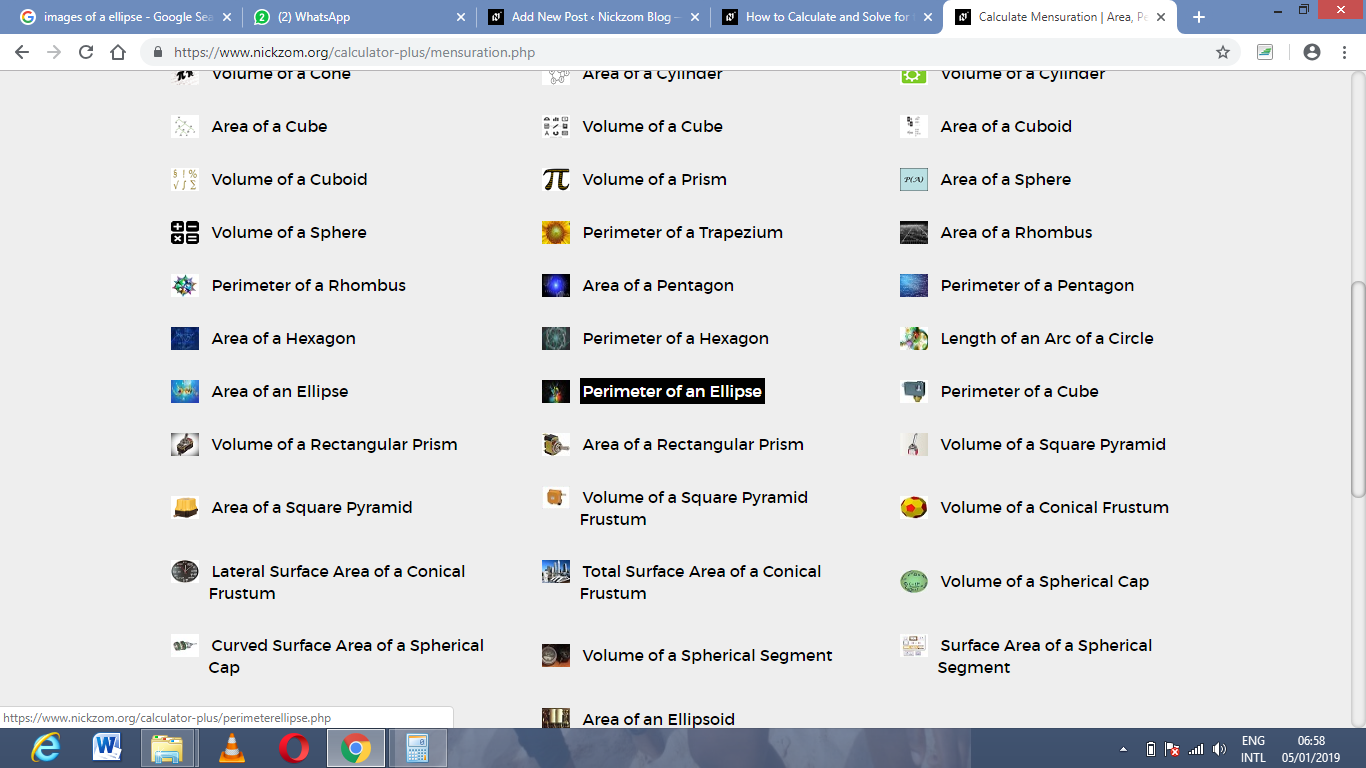# How to Calculate and Solve for the Perimeter of an Ellipse | Nickzom CalculatorThe image above is an ellipse.

To compute the perimeter of an ellipse, two essential parameters are needed and this parameters are axis (a) and axis (b).

The formula for calculating the perimeter of an ellipse:

P = π [3(a + b) – √((3a + b)(a + 3b))]

Where;

P = Perimeter of the ellipse
a = Axis of the ellipse
b = Axis of the ellipse

Let’s solve an example;
Given that the axis of the ellipse (a) is 19 cm and axis of the ellipse (b) is 31 cm. Find the perimeter of the ellipse?

This implies that;
a = Axis of the ellipse = 19 cm
b = Axis of the ellipse = 31 cm

P = π [3(a + b) – √((3a + b)(a + 3b))]
P = 3.142 [3(19 + 31) – √((3 x 19 + 31)(19 + 3 x 31))]
P = 3.142 [3(50) – √((57+ 31)(19 + 93))]
P = 3.142 [150 – √((88)(112))]
P = 3.142 [150 – √(9856)]
P = 3.142 [150 – 99.28]
P = 3.142 [50.72]
P = 3.142 x 50.72
P = 159.26

Therefore, the perimeter of the ellipse is 159.26 cm.

Nickzom Calculator – The Calculator Encyclopedia is capable of calculating the perimeter of an ellipse.

To get the answer and workings of the perimeter of an ellipse using the Nickzom Calculator – The Calculator Encyclopedia. First, you need to obtain the app.

You can get this app via any of these means:

To get access to the professional version via web, you need to register and subscribe for NGN 1,500 per annum to have utter access to all functionalities.
You can also try the demo version via https://www.nickzom.org/calculator

Once, you have obtained the calculator encyclopedia app, proceed to the Calculator Map, then click on Mensuration under the Mathematics sectionNow, click on Perimeter of an Ellipse under MensurationThe screenshot below displays the page or activity to enter your values, to get the answer for the perimeter of an ellipse according to the respective parameters which are the axis (a) and axis (b) of the ellipse.Now, enter the values appropriately and accordingly for the parameters as required by the example above where the axis (a) is 19 cm and axis (b) is 31 cm.Finally, Click on CalculateAs you can see from the screenshot above, Nickzom Calculator – The Calculator Encyclopedia solves for the perimeter of an ellipse and presents the formula, workings and steps too.